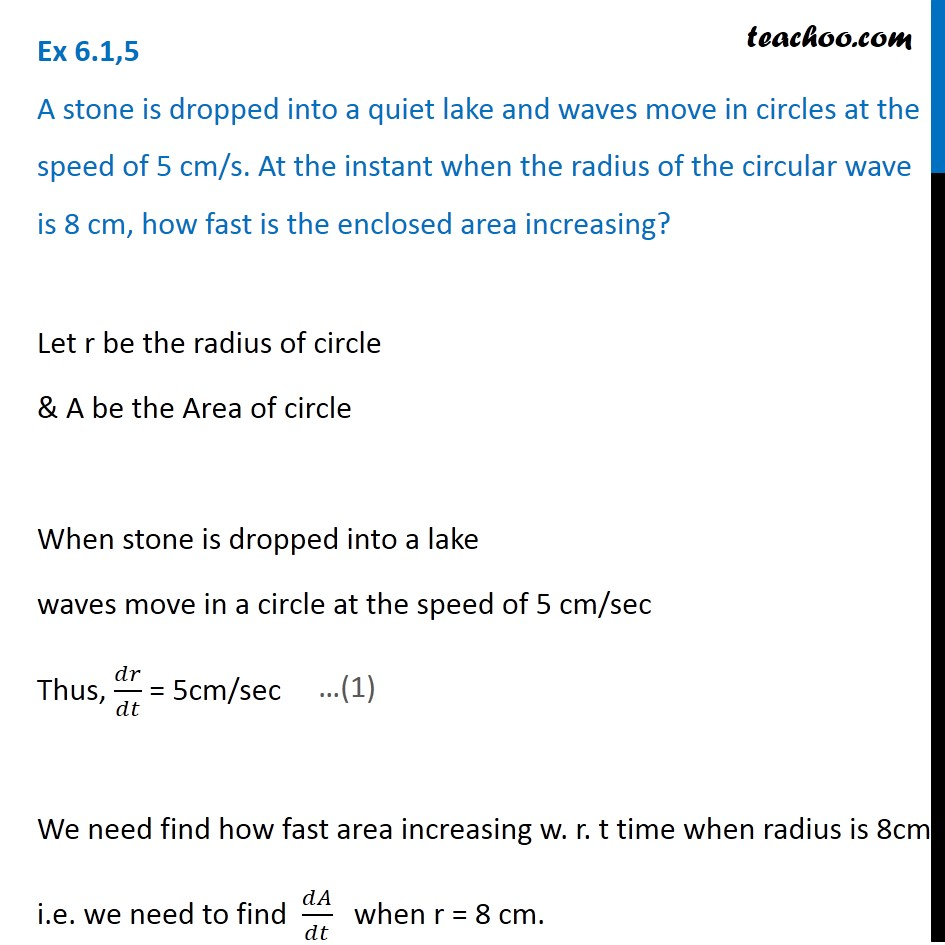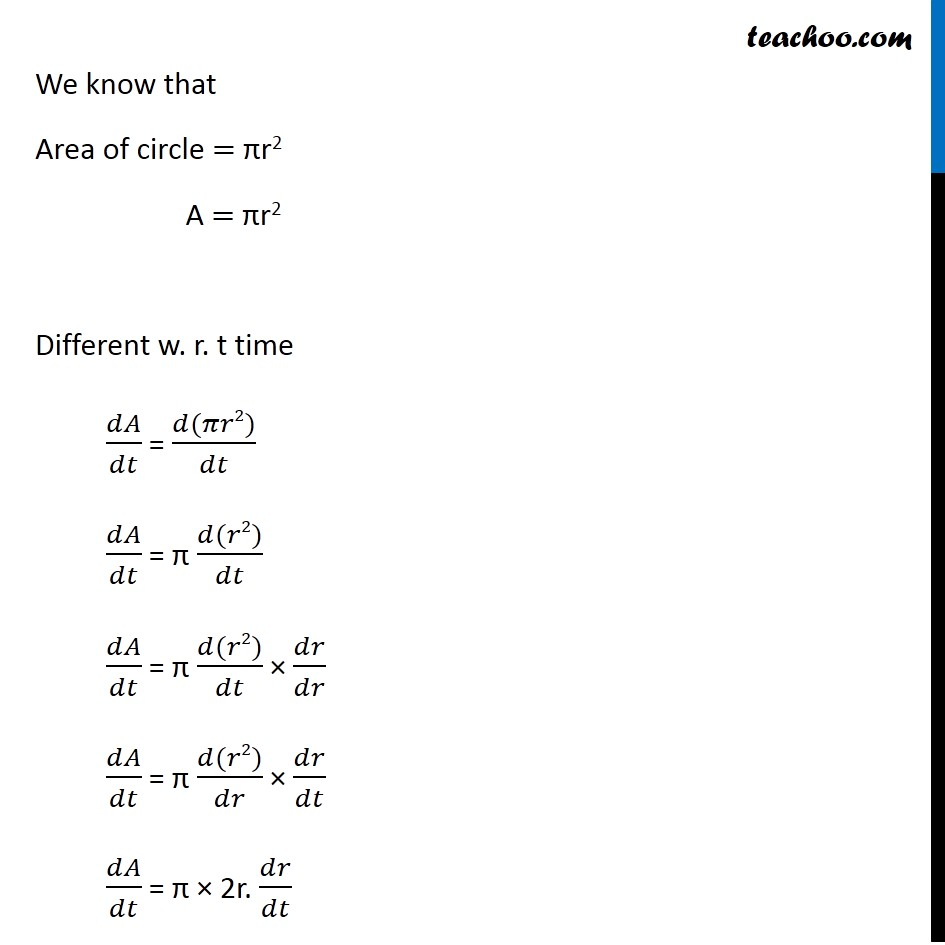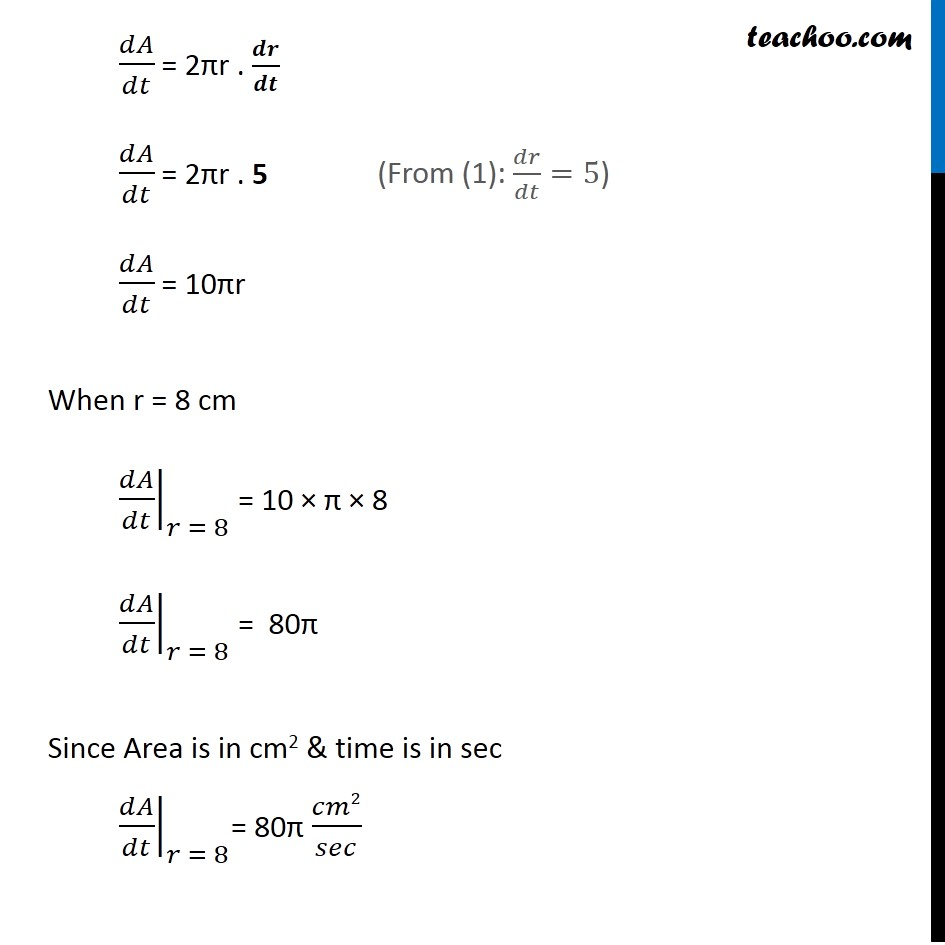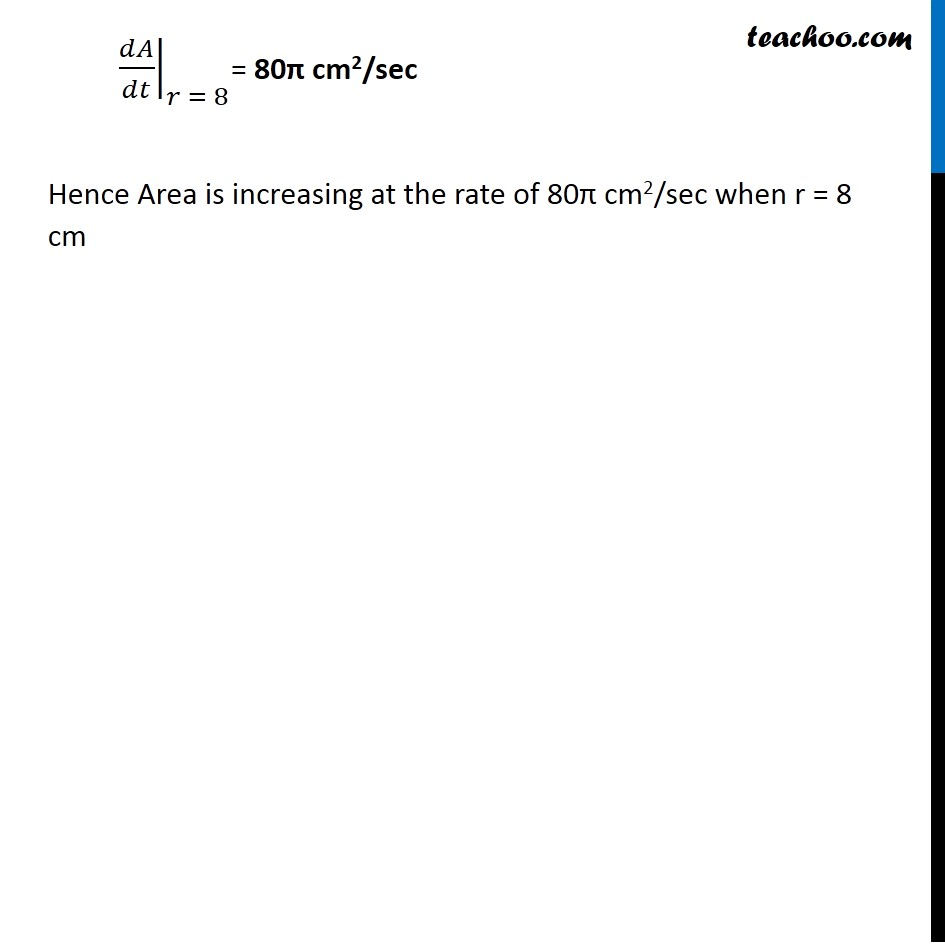Check Full Chapter Explained - Continuity and Differentiability - Application of Derivatives (AOD) Class 121. Chapter 6 Class 12 Application of Derivatives
2. Serial order wise
3. Ex 6.1

Transcript

Ex 6.1,5 A stone is dropped into a quiet lake and waves move in circles at the speed of 5 cm/s. At the instant when the radius of the circular wave is 8 cm, how fast is the enclosed area increasing? Let r be the radius of circle & A be the Area of circle When stone is dropped into a lake waves move in a circle at the speed of 5 cm/sec Thus, 𝑑𝑟/𝑑𝑡 = 5cm/sec We need find how fast area increasing w. r. t time when radius is 8cm i.e. we need to find 𝑑𝐴/𝑑𝑡 when r = 8 cm. We know that Area of circle = πr2 A = πr2 Different w. r. t time 𝑑𝐴/𝑑𝑡 = (𝑑(𝜋𝑟2))/𝑑𝑡 𝑑𝐴/𝑑𝑡 = π (𝑑(𝑟2))/𝑑𝑡 𝑑𝐴/𝑑𝑡 = π (𝑑(𝑟2))/𝑑𝑡 × 𝑑𝑟/𝑑𝑟 𝑑𝐴/𝑑𝑡 = π (𝑑(𝑟2))/𝑑𝑟 × 𝑑𝑟/𝑑𝑡 𝑑𝐴/𝑑𝑡 = π × 2r. 𝑑𝑟/𝑑𝑡 𝑑𝐴/𝑑𝑡 = 2πr . 𝒅𝒓/𝒅𝒕 𝑑𝐴/𝑑𝑡 = 2πr . 5 𝑑𝐴/𝑑𝑡 = 10πr When r = 8 cm ├ 𝑑𝐴/𝑑𝑡┤|_(𝑟 = 8) = 10 × π × 8 ├ 𝑑𝐴/𝑑𝑡┤|_(𝑟 = 8) = 80π Since Area is in cm2 & time is in sec ├ 𝑑𝐴/𝑑𝑡┤|_(𝑟 = 8)= 80π 𝑐𝑚2/𝑠𝑒𝑐 (From (1): 𝑑𝑟/𝑑𝑡=5) ├ 𝑑𝐴/𝑑𝑡┤|_(𝑟 = 8)= 80π cm2/sec Hence Area is increasing at the rate of 80π cm2/sec when r = 8 cm

Ex 6.1